# NCERT Solutions Class 8 Science Stars and the Solar System

## Stars and the Solar System Class 8 NCERT Solutions

Class 8 Science students should refer to the following NCERT questions with answers for Stars and the Solar System in standard 8. These NCERT Solutions with answers for Grade 8 Science will come in exams and help you to score good marks

### Stars and the Solar System NCERT Solutions Class 8

NCERT Solutions for Class 8 Science for chapter 17 Stars and the Solar System

1. Which of the following is NOT a member of the solar system?

(a) An asteroid

(b) A satellite

(c) A constellation

(d) A comet

Answer: The correct option is (c).

Explanation: A constellation is the group of stars which forms recognisable shapes. Hence, it is not a member of the solar system.

2. Which of the following is NOT a planet of the sun?

(a) Sirius (b) Mercury (c) Saturn (d) Earth

Answer: The correct option is (a).

Explanation: Sirius is a star and not a planet of the Sun.

3. Phases of the moon occur because

(a) we can see only that part of the moon which reflects light towards us.

(b) our distance from the moon keeps changing.

(c) the shadow of the Earth covers only a part of the moon’s surface.

(d) the thickness of the moon’s atmosphere is not constant.

Answer: The correct option is (a).

Explanation: Phases of the moon are seen because we can see only that part of the moon which reflects light towards us.

4. Fill in the blanks:

(a) The planet which is farthest from the sun is______.

(b) The planet which appears reddish in colour is ______.

(c) A group of stars that appear to form a pattern in the sky is known as a ______.

(d) A celestial body that revolves around a planet is known as a _____.

(e) Shooting stars are actually not______

(f) Asteroids are found between the orbits of ____and _____.

(a) The planet which is farthest from the Sun is Neptune.

(b) The planet which appears reddish in colour is Mars.

(c) A group of stars that appear to form a pattern in the sky is known as a constellation.

(d) A celestial body that revolves around a planet is known as a satellite.

(e) Shooting stars are actually not stars.

(f) Asteroids are found between the orbits of Mars and Jupiter.

5. Mark the following statement as true (T) or false (F).

(a) Pole star is a member of the solar system. ( )

(b) Mercury is the smallest planet of the solar system. ( )

(c) Uranus is the farthest planet in the solar system. ( )

(d) INSAT is an artificial satellite. ( )

(e) There are nine planets in the solar system. ( )

(f) Constellation Orion can be seen only with a telescope. ( )

(a) Pole star is a member of the solar system. (False )

Explanation: Stars are not the member of the solar system. Only Sun and the celestial bodies which are revolving around the Sun are the parts of the solar system.

(b) Mercury is the smallest planet of the solar system. (True)

(c) Uranus is the farthest planet in the solar system. (False)

Explanation: Neptune is the farthest planet in the solar system.

(d) INSAT is an artificial satellite. (True)

(e) There are nine planets in the solar system. (False)

Explanation: There are eight planets in the solar system.

(f) Constellation Orion can be seen only with a telescope. (False)

Explanation: Orion can be seen during winters around late evenings. It is one of the most magnificent constellations in the sky and can be seen by naked eyes.

6. Match items in column A with one or more items in column B.

 A B (i) Inner Planets (a) Saturn (ii) Outer Planets (b) Pole Star (iii) Constellation (c) Great Bear (iv) Satellite of the Earth (d) Moon (e) Earth (f) Orion (g) Mars

 A B (i) Inner Planets (g), (e) Mars, Earth (ii) Outer Planets (a) Saturn (iii) Constellation (c), (f) Great Bear, Orion (iv) Satellite of the earth (d) Moon

7. In which part of the sky can you find Venus if it is visible as an evening star?

Answer: Venus (evening star) can be seen in the western sky after sunset.

8. Name the largest planet of the solar system.

Answer: Jupiter is the largest planet of the solar system.

9. What is a constellation? Name any two constellations.

Answer: A constellation is a group of stars that forms a recognisable pattern in the sky. The two very important constellations are Ursa Major and Orion.

10. Draw sketches to show the relative position of prominent stars in (a) Ursa Major and (b) Orion

(a) Ursa Major: It looks like a big dipper. There are three bright stars in the handle and four stars in the bowl of the dipper.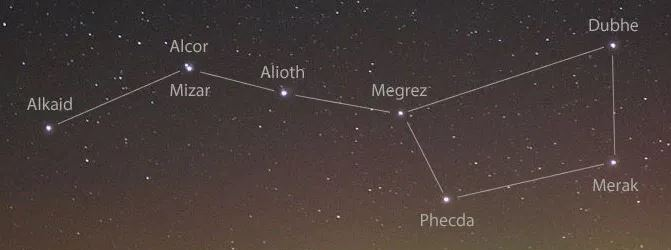(b) Orion: It looks like a hunter. Three bright stars appear in the belt, while five bright stars are arranged in the form of a quadrilateral.11. Name two objects other than planets which are members of the solar system.

(i) Asteroids: These are the collection of a large number of small objects, gases and dust revolving around the Sun. They cover a large gap between the orbits of Mars and Jupiter.

(ii) Meteors: They are small celestial objects that are seen as bright streaks of light in the sky. They burnt out on entering the Earth’s atmosphere because of the heat produced by friction. This results in bright streaks in the sky.

12. Explain how you can locate the Pole Star with the help of Ursa Major.

The Pole star can be located with the help of Big Dipper constellation. In Big Dipper constellation, the bowl has four bright stars. Now, trace an imaginary straight line towards the Northern direction connecting two stars at the end of this bowl. This imaginary dotted line joins to a star known as the Pole Star.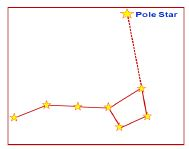13. Do all the stars in the sky move? Explain.

Answer: The stars do not move in the sky. However, the stars seem to move in the sky for an observer on the Earth. It is because the Earth rotates from west to east on its axis. Hence, all the stars in the sky appear to move from east to west except Pole star as it is located above the axis of rotation of the Earth in the north direction.

14. Why is the distance between stars expressed in light years? What do you understand by the statement that a star is eight light years away from the Earth?

Answer: The distance between stars or distance of a star from the Earth is so large that it is inconvenient to express these distances in kilometers. Therefore, these large distances are measured in light years. One light year is equal to the distance travelled by light in one year and is equal to 9.46 × 1012 km.

If a star is located eight light years away from the Earth, it means distance between the star and the Earth is = 8 × (9.46 × 1012) km or 7.6 × 1013 km away from the Earth.

15. The radius of Jupiter is 11 times the radius of the Earth. Calculate the ratio of the volumes of Jupiter and the Earth. How many Earths can Jupiter accommodate?

Earth and Jupiter can be assumed as two spheres. Let, radus of the Earth = r1

radius of the Jupiter = r2

Hence, r2 = 11 r1

Volume (V) of a sphere of radius r is given as,

V = 4/3 πr3

∴ Volume of Earth, V= 4/3 πr13   ... (i)

Volume of Jupiter, V2 = 4/3 πr2 3   = 4/3 π (11 r1 )... (ii)

On dividing equation (ii) by (i),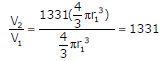or, V2 = 1331 V1

Hence, Jupiter can accommodate 1331 Earths.

16. Boojho made the following sketch (Fig. 17.29) of the solar system. Is the sketch correct? If not, correct it.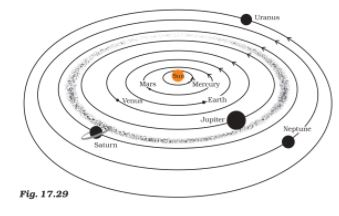Answer: The sequence of the planets in the solar system from the Sun is: Mercury, Venus, Earth, Mars, Jupiter, Saturn, Uranus and Neptune. Hence, the sequence of the planets of solar system is not correctly sketched by Boojho. Moreover, the asteroid belt should be located between the orbits of Mars and Jupiter. The correct sketch of the solar system is shown in the figure given below.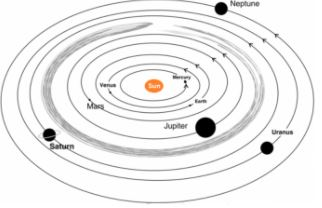## Books recommended by teachers

 NCERT Solutions Class 8 Science Crop production and management
 NCERT Solutions Class 8 Science Microorganisms Friends and Foe
 NCERT Solutions Class 8 Science Synthetic fibres and plastics
 NCERT Solutions Class 8 Science Materials Metals and Non-Metals
 NCERT Solutions Class 8 Science Coal and Petroleum
 NCERT Solutions Class 8 Science Combustion and Flame
 NCERT Solutions Class 8 Science Conservation of Plants and Animals
 NCERT Solutions Class 8 Science Cell Structure and Functions
 NCERT Solutions Class 8 Science Reproduction in Animals
 NCERT Solutions Class 8 Science Reaching the Age of Adolescence
 NCERT Solutions Class 8 Science Force and Pressure
 NCERT Solutions Class 8 Science Friction
 NCERT Solutions Class 8 Science Sound
 NCERT Solutions Class 8 Science Chemical Effects of Electric Current
 NCERT Solutions Class 8 Science Some Natural Phenomena
 NCERT Solutions Class 8 Science Light
 NCERT Solutions Class 8 Science Stars and the Solar System
 NCERT Solutions Class 8 Science Pollution of Air and Water